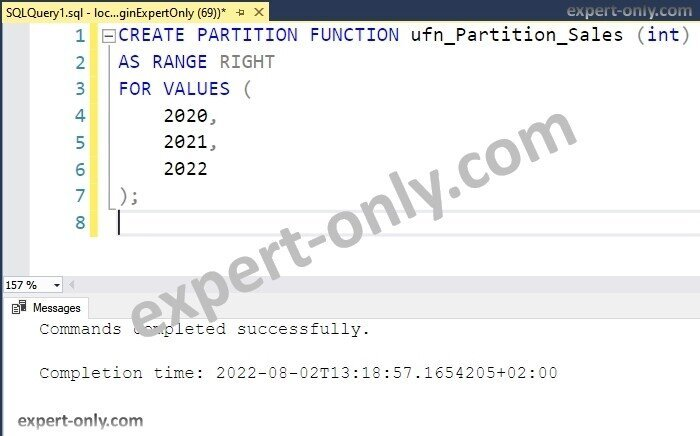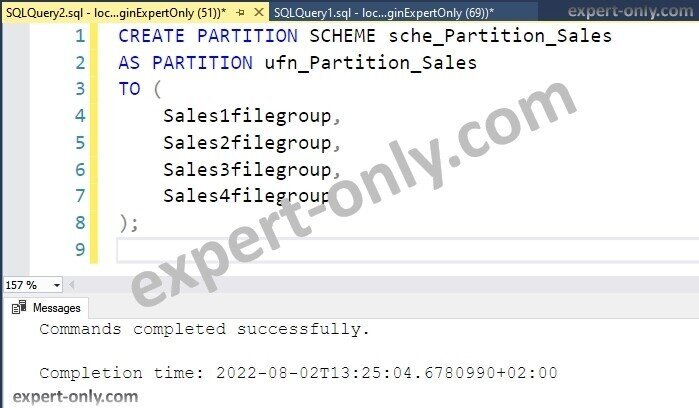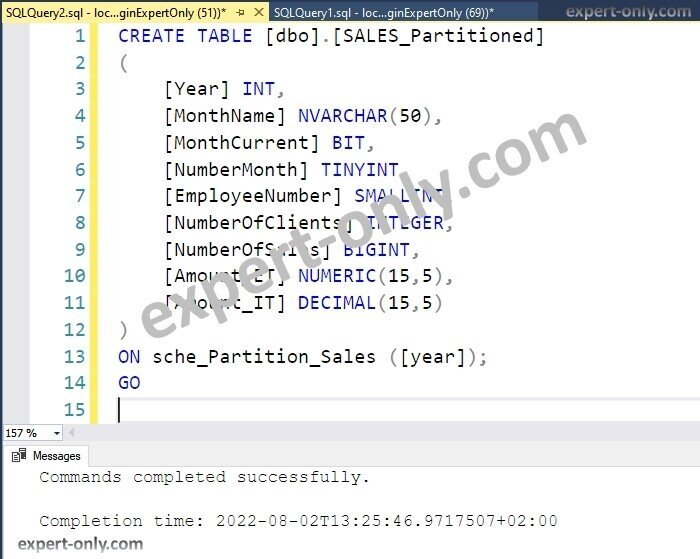# Create a SQL Server partition

How to create a table partition in SQL Server? Let’s consider a large table with Sales data. So, this table have a column that stores the year of the sale and the table stores millions of lines.

Let’s consider also that some business reports read this data to display yearly totals and compares them to the previous year totals. It is called a year over year comparison.

## Scripts to create a SQL Server partition step by step

To start, let’s use this sales table as an example for the partition creation.

```CREATE TABLE [dbo].[SALES]
(
[Year] INT,
[MonthName] NVARCHAR(50), -- alphanumeric
[MonthCurrent] BIT, -- Boolean, 0 or 1 , false / true
[NumberMonth] TINYINT, -- very small integer, from 0 to 255
[EmployeeNumber] SMALLINT, -- small integer, minimum -2^15, maximum 2^15
[NumberOfClients] INTEGER, -- integer, minimum -2^31, maximum 2^31
[NumberOfSales] BIGINT, -- big integer, minimum: -2^63, maximum 2^63
[Amount_ET] NUMERIC(15,5), -- numeric, 15 digits, with 5 after the comma
[Amount_IT] DECIMAL(15,5) -- decimal, 15 digits, with 5 after the comma
);
GO
```

To clarify, the partitioning of a table happens with four main objects and decisions to take. The main decision is to choose the partitioning column.

### 1. First step is to choose the partitioning column

First, let’s choose the best column for the partition. As the article exposes earlier in the introduction, many queries use the year as a filter to display yearly figures and year over year comparisons.
The choice of a partition on the year column is an interesting one. The partitioning column is the Year column and it’s an integer.

The different partitions on the year will split physically the data in the disk on multiple file groups. This operation is also called partitioning an existing table. Check more details in this article on the definition of a SQL Server partition.

Moreover, the table remains one unique logical SQL Server object, but physically it’s split in different files to isolate and improve the access to every partition.

### 2. Second step is to create a partition function

Secondly, to create a partition function, simply use a CREATE statement in order to create the partition function. The parameters are the data type of the partitioning column, the type of partition than can be right or left, and the range of values.

For instance, this T-SQL code example shows how to create a partition function based on the previous years, the last year, the current year and the next years.

```CREATE PARTITION FUNCTION ufn_Partition_Sales (int)
AS RANGE RIGHT
FOR VALUES (
2018,
2019,
2020
);```T-SQL script to create a partition function with range right option as partition scheme

### 3. Third step is to create the necessary file groups used by the SQL partitions

Moreover, a partition scheme uses a partition function, and a partition scheme uses file groups. To create the partition scheme, create first the file groups to be used.

Above all, make sure to adjust the code before running it. Use the code below to create four additional file groups to the existing Expert-Only database. After that, the next example of code creates the file groups in the file system. In addition, check out the official documentation on partitioned tables and indexes.

```USE [Expert-Only];
GO

ALTER DATABASE [Expert-Only] ADD FILEGROUP Sales1filegroup;
GO

ALTER DATABASE [Expert-Only] ADD FILEGROUP Sales2filegroup;
GO

ALTER DATABASE [Expert-Only] ADD FILEGROUP Sales3filegroup;
GO

ALTER DATABASE [Expert-Only] ADD FILEGROUP Sales4filegroup;
GO
```

In addition, the next step is mandatory and assigns the physical files to existing file groups. To do this, let’s add a secondary data file to every file group created.

```ALTER DATABASE [Expert-Only] ADD FILE
(
NAME = Sales1datafile,
FILENAME =
'C:\Program Files\Microsoft SQL Server\MSSQL15.MSSQLSERVER\MSSQL\DATA\Sales1df.ndf',
SIZE = 10MB, MAXSIZE = 500MB, FILEGROWTH = 10MB
)
TO FILEGROUP Sales1filegroup;
GO

ALTER DATABASE [Expert-Only] ADD FILE
(
NAME = Sales2datafile,
FILENAME =
'C:\Program Files\Microsoft SQL Server\MSSQL15.MSSQLSERVER\MSSQL\DATA\Sales2df.ndf',
SIZE = 10MB, MAXSIZE = 500MB, FILEGROWTH = 10MB
)
TO FILEGROUP Sales2filegroup;
GO

ALTER DATABASE [Expert-Only] ADD FILE
(
NAME = Sales3datafile,
FILENAME =
'C:\Program Files\Microsoft SQL Server\MSSQL15.MSSQLSERVER\MSSQL\DATA\Sales3df.ndf',
SIZE = 10MB, MAXSIZE = 500MB, FILEGROWTH = 10MB
)
TO FILEGROUP Sales3filegroup;
GO

ALTER DATABASE [Expert-Only] ADD FILE
(
NAME = Sales4datafile,
FILENAME =
'C:\Program Files\Microsoft SQL Server\MSSQL15.MSSQLSERVER\MSSQL\DATA\Sales4df.ndf',
SIZE = 10MB, MAXSIZE = 500MB, FILEGROWTH = 10MB
)
TO FILEGROUP Sales4filegroup;
GO
```

### 4. Fourth step is to create a SQL Server partition scheme

Further, the partition scheme object defines how to spread the data in the different file groups. Hence improving performance and maintenance of the table.

```CREATE PARTITION SCHEME sche_Partition_Sales
AS PARTITION ufn_Partition_Sales
TO (
Sales1filegroup,
Sales2filegroup,
Sales3filegroup,
Sales4filegroup
);```Add a SQL Server partition scheme and use the four data file groups for the sales table

### 5. Update the existing table or create a new one to use the partition scheme

Finally, the table is partitioned after using the partition scheme and the partition function. The code creates the partitions for the newly created Sales table. It also passes the year as the partitioning column.

```CREATE TABLE [dbo].[SALES_Partitioned]
(
[Year] INT,
[MonthName] NVARCHAR(50),
[MonthCurrent] BIT,
[NumberMonth] TINYINT,
[EmployeeNumber] SMALLINT,
[NumberOfClients] INTEGER,
[NumberOfSales] BIGINT,
[Amount_ET] NUMERIC(15,5),
[Amount_IT] DECIMAL(15,5)
)
ON sche_Partition_Sales ([year]);
GO
```Script in T-SQL to create a table with partitions by year

## Insert data in the newly created table partition

To insert data in the newly created table using partitions, is it is simple. The storage is managed by the partition function and a simple Insert statement works as usual.

The goal is to insert data for multiple years and check in which partition the data is stored.

```INSERT INTO [dbo].[SALES_Partitioned] ([Year], [MonthName], [Amount_ET]) values (2017, 'January', 10000);
INSERT INTO [dbo].[SALES_Partitioned] ([Year], [MonthName], [Amount_ET]) values (2018, 'January', 11000);
INSERT INTO [dbo].[SALES_Partitioned] ([Year], [MonthName], [Amount_ET]) values (2019, 'January', 12000);
INSERT INTO [dbo].[SALES_Partitioned] ([Year], [MonthName], [Amount_ET]) values (2020, 'January', 13000);
INSERT INTO [dbo].[SALES_Partitioned] ([Year], [MonthName], [Amount_ET]) values (2021, 'January', 14000);
INSERT INTO [dbo].[SALES_Partitioned] ([Year], [MonthName], [Amount_ET]) values (2022, 'January', 15000);
INSERT INTO [dbo].[SALES_Partitioned] ([Year], [MonthName], [Amount_ET]) values (2023, 'January', 16000);
```

After inserting some values for multiples years, from 2017 to 2023, let’s check in which partition the data is stored. In fact, we can list in which filegroup the Sales data is stored, and how many lines are present.

To achieve this, we use mainly two system views: the partitions system view and the dm_db_partition_stats that displays statistics on partitions.

```select
object_name(ps.object_id) as TableName,
ps.partition_number as PartitionNumber,
fg.name AS FileGroupName,
row_count as [RowCount]
from	sys.dm_db_partition_stats ps,
sys.partitions p
join sys.allocation_units au
ON au.container_id = p.hobt_id
join sys.filegroups fg
ON fg.data_space_id = au.data_space_id
where	p.partition_id = ps.partition_id
and		ps.[object_id] in (
select object_id('[dbo].[SALES]')
union all
select object_id('[dbo].[SALES_Partitioned]')
)
order by 2;```SQL Server query to display table and file group names with number of rows

In the example, the data is stored this way by the database system, because we have selected the RANGE RIGHT option in the Partition Function :

1. Sales1filegroup stores the data with year strictly inferior to 2020 (etc., 2017,2018,2019)
2. Sales2filegroup stores the data equal or larger than 2020 and inferior to 2021 (2020)
3. Sales3filegroup contains the data equal or larger than 2021 and inferior to 2022 (2021)
4. Sales4filegroup contains all the data superior or equal to 2022 (2022, 2023, etc…)

To conclude, this article explains how to create a SQL Server partition table with a partitioning column, a partition function, and a partition scheme.

What is a SQL Server table partition?

A partition table is a MS SQL table with a partitioning column, it uses a partition function and a partition scheme. In addition, it contains data that is split in the disk to access directly to one single piece of data instead of accessing the full rows of a unique table.

How to create a table partition by year?

A table partition by year uses the year as a partitioning column and stores the data for a given year in a specific partition. In other words, the general goal is to have the most frequently used years, like the last year, the current year, and the next year in dedicated separate partitions.

How to improve SQL Server query performance?

To improve the performance of MSSQL queries, creating a table partition and adding indexes help a lot. Moreover, the partitioning helps to physically split the data into different pieces making the system respond faster.

What are the disadvantages of SQL Server partitions?

The main disadvantages of MS SQL table partitions are the object maintenance to keep the partition definitions up to date with the partitioning column.

To go further, let’s check out how to create a view with MSSQL to group data from multiple tables.